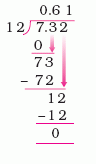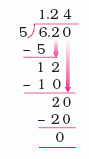Email us to get an instant 20% discount on highly effective K-12 Math & English kwizNET Programs!

#### Online Quiz (WorksheetABCD)

Questions Per Quiz = 2 4 6 8 10

### Grade 5 - Mathematics4.1 Dividing Decimals by Whole Numbers

 Division of Decimals by whole number: Method: To divide a decimal by a whole number, we divide the whole number part of the dividend first. After that a decimal is placed and the decimal part of the dividend is divided. When the whole number part of the dividend is less than the divisor, we first place a 0 followed by the decimal and the dividend is divided as usual. Example 1:Look at the dividend, start with the leftmost digit Since 7 < 12, the divisor 12 goes 0 times into 7. We place 0 in the quotient in the ones place. We then put a decimal point at the right of 0 in the quotient and divide as usual. Answer: 0.61 Example 2:Divide 6 by 5 and the remainder is 1, then decimal point is put in the quotient after 1. Bring the next digit 2 down, divide 12 by 5, the remainder is 2. To divide the last remainder 2, we put a 0 at the end of the dividend and the remainder to get 20. 20 divided by 5 gives 4 and the remainder is 0. Answer: 1.24 Directions: Divide the following. Also write at least 5 examples of your own.
 Q 1: 0.18 / 120.0150.0190.0109 Q 2: 0.085 / 50.0150.0170.016 Q 3: 18.27 / 92.042.052.03 Q 4: 73.836 / 184.1004.1024 Q 5: 91.56 / 422.182.142.16 Q 6: 3.93 / 31.311.331.30 Q 7: \$8.64 / 3\$2.00\$2.88\$3.88 Q 8: 57.6 / 321.81.61.7 Question 9: This question is available to subscribers only! Question 10: This question is available to subscribers only!